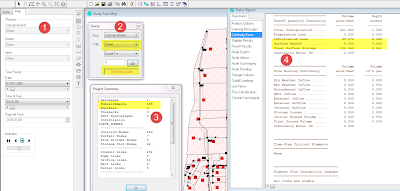## Sunday, January 19, 2014

### How SWMM5 uses zero Subcatchment Slope in its Runoff Calculations

This is just a note on how SWMM5 uses zero Subcatchment Slope in its Runoff Calculations:
1. If the slope is zero then the engine will NOT calculate Runoff as the WCON parameter is zero (Bullet 4 in Figure 1)
2. If you do want Runoff from all of your Subcatchments then you need to check the slope value in your Hydrology by doing a data query (Bullet 2 in Figure 2)
3. If you want to use the zero slope as a facility manager in your models then you can use a zero slope and have no Runoff. it is a way to deactivate a portion or section of your model.Figure 1.  How SWMM5 Uses Zero Subcatchment Slope